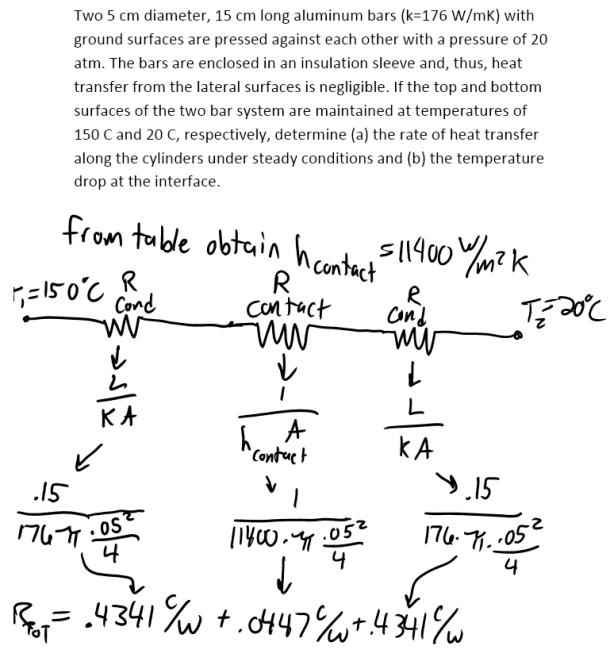heat and mass transfer problems engineering equations heat and mass transfer school homework engineering heat and mass transfer formulas heat and mass transfer solutions to heat and mass transfer problems full solution engineering problem solution heat and mass transfer math problems engineering equations heat and mass transfer school homework engineering solutions to heat and mass transfer formulas heat problems mass problem solutions to transfer problems full solution heat and mass transfer
heat and mass transfer problems engineering equations heat and mass transfer school homework engineering heat and mass transfer formulas heat and mass transfer solutions to heat and mass transfer problems full solution engineering problem solution heat and mass transfer math problems engineering equations heat and mass transfer school homework engineering solutions to heat and mass transfer formulas heat problems mass problem solutions to transfer problems full solution heat and mass transfer
Highalphabet Home Page heat and mass transfer problem solutions Heat and Mass Transfer Page
Two 5 cm diameter, 15 cm long aluminum bars (k=176 W/mK) with ground surfaces are pressed against each other with a pressure of 20 atm. The bars are enclosed in an insulation sleeve and, thus, heat transfer from the lateral surfaces is negligible. If the top and bottom surfaces of the two bar system are maintained at temperatures of 150 C and 20 C, respectively, determine (a) the rate of heat transfer along the cylinders under steady conditions and (b) the temperature drop at the interface.Two 5 cm diameter, 15 cm long aluminum bars (k=176 W/mK) with ground surfaces are pressed against each other with a pressure of 20 atm. The bars are enclosed in an insulation sleeve and, thus, heat transfer from the lateral surfaces is negligible. If the top and bottom surfaces of the two bar system are maintained at temperatures of 150 C and 20 C, respectively, determine (a) the rate of heat transfer along the cylinders under steady conditions and (b) the temperature drop at the interface.